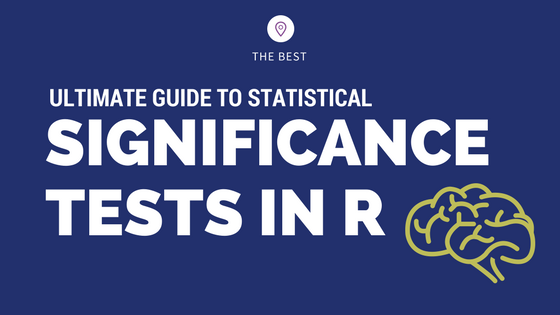## How to implement common statistical significance tests and find the p value?

How to implement and interpret the commonly used statistical significance tests in R? Understand the purpose, when to use and how to interpret the test results and the p value. Correlation Test and Introduction to p value One Sample t-Test Wilcoxon Signed Rank Test Two Sample t-Test and Wilcoxon Rank Sum Test Shapiro Test Kolmogorov …